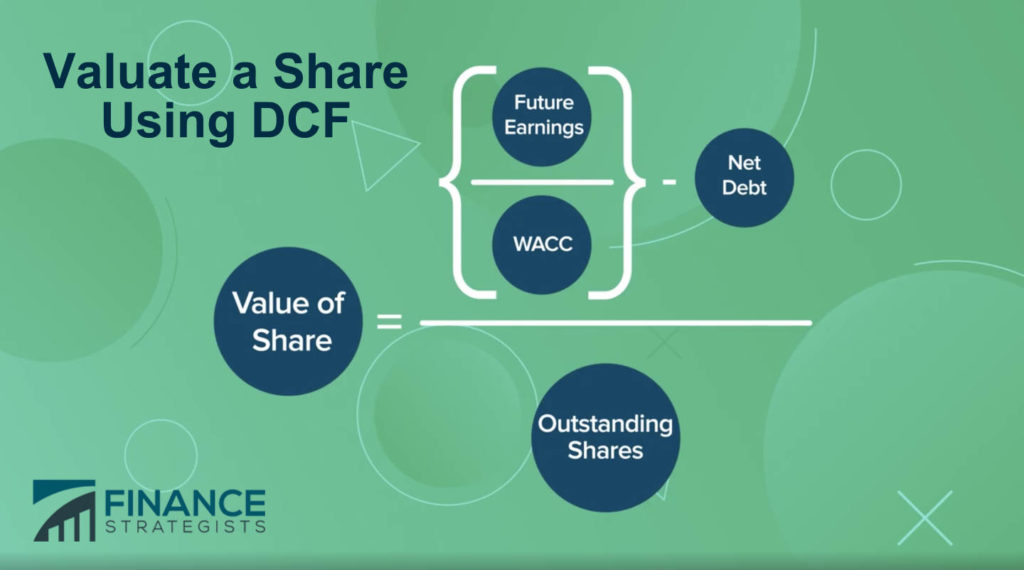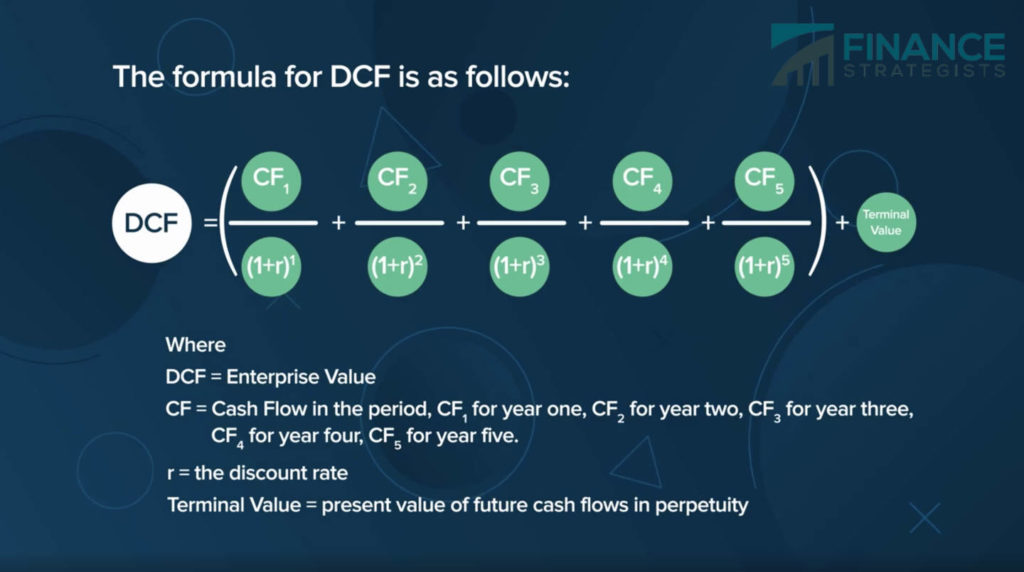# Discounted Cash Flow Model (DCF) Definition

Discounted Cash Flow Model, or DCF Model, values a company based on its future cash flows discounted by the investor’s expected rate of return, which is then used to calculate the stock price.

The Discounted Cash Flow Model can be used even if a company doesn’t pay a dividend or has unpredictable dividend returns.

## DCF Valuation

To calculate the value of a share using the Discounted Cash Flow Model, add up the value of future earnings, then discount the earnings by the weighted average cost of capital, or WACC.

Then, subtract net debt from the enterprise value to compute the company’s fair value and divide by the number of outstanding shares to calculate the value of a share.## Discounted Cash Flow Formula

The formula for DCF is as follows:

Take the future cash flows for year.

Then divide by one plus “r”, which represents the discount rate, or WACC, raised to the first power.

Then, add the future cash flows for year 2.

Divided by one plus “r”, or the WACC, raised to the second power.

And continue this sequence using a detailed forecast for five years, then add a terminal value, which is the present value of future cash flows in perpetuity.

Once you have the enterprise value, subtract the net debt of the company and divide by the number of outstanding shares.## Discounted Cash Flow Analysis

If the estimated value of a share using the Discounted Cash Flow Model is greater than the current value of a share, the DCF model suggests it is a buying opportunity.

The weakness of this model is that it relies entirely on future cash flows estimates, which are unknown.

Additionally, appraising the cash flows and discount rates incorrectly can lead to inaccurate conclusions on the attractiveness of the investment.

Learn the basics of Cash Flow Statements, their objectives, and classifications in this article: Cash Flow Statement (CFS)

## Discounted Cash Flow FAQs

DCF stands for the discounted cash flow model.
Discounted Cash Flow Model, or DCF Model, values a company based on its future cash flows discounted by the investor’s expected rate of return, which is then used to calculate the stock price.
The Discounted Cash Flow Model can be used even if a company doesn’t pay a dividend or has unpredictable dividend returns.
To calculate the value of a share using the Discounted Cash Flow Model, add up the value of future earnings, then discount the earnings by the weighted average cost of capital, or WACC.

## About the AuthorTrue Tamplin, BSc, CEPF®

True Tamplin is a published author, public speaker, CEO of UpDigital, and founder of Finance Strategists.

True is a Certified Educator in Personal Finance (CEPF®), a member of the Society for Advancing Business Editing and Writing, contributes to his financial education site, Finance Strategists, and has spoken to various financial communities such as the CFA Institute, as well as university students like his Alma mater, Biola University, where he received a bachelor of science in business and data analytics.

To learn more about True, visit his personal website, view his author profile on Amazon, or check out his speaker profile on the CFA Institute website.